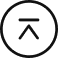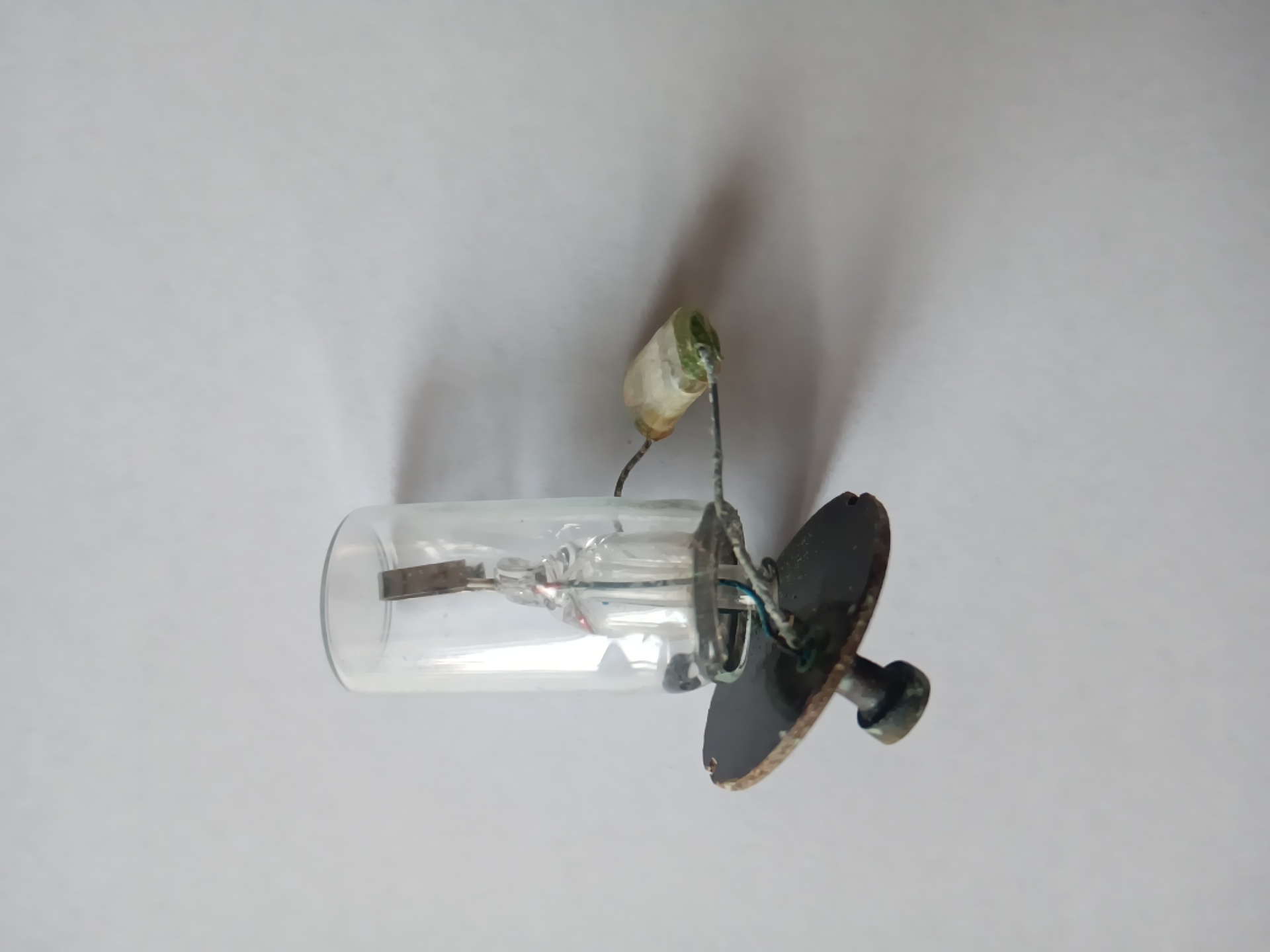回到顶部

# 【跨学科电学案例二】电容器的电容 中等

教学目标：1.知道什么是电容器及电容器的主要构造。

2.掌握电容的概念及其定义式，并利用定义式进行简单计算。（重点）

3.了解电容器充电和放电现象,分析电荷量变化及能量转化情况。（难点）

作为本节难点，通过普通电压表来观察电容器的充放电现象，其实难度是不小的，一是演示实验一中，比较难看到电压与时间的函数关系，二是演示实验二中由于电容器非常容易漏电，较难看到B电容器电压是A电容器电压的一半，而笔者通过行空板能较好地解决利用传统电压表所带来的一些问题。

2.软件部分：可以使用Mind+对行空板进行编程，编程主要分为数据采集和数据处理、复位三部分。考虑到由于一般的电容器充放电时间很短，设备采集较为困难。所以在电路设计时候采用加大电阻延长充放电的时间的方法，而大电阻又会导致电流过小而无法采集，所以这里主要采用采集电压的方式来进行程序的设计。（具体程序可以下载附件）

from matplotlib import pyplot as plt
import matplotlib

from pinpong.libs.dfrobot_ina219 import INA219
from pinpong.extension.unihiker import *
from pinpong.board import Board,Pin
from pinpong.board import Board
from unihiker import GUI

# 事件回调函数
global B
global t
global x
global y
global t
while True:
if (button_a.is_pressed()==True):
B = (B + 1)
if (B == 1):
P=u_gui.draw_text(text="数据处理中",x=80,y=120,font_size=20, color="#0000FF")
elif (button_b.is_pressed()==True):
plt.close()
B = 0
t = 0
x = []
y = []
P.config(text="")

Board().begin()
u_gui=GUI()
global t
global x
global y
global B
B = 0
global t
t = 0
x = []
y = []
ina1.begin()
u_gui.draw_text(text="电容器电压",x=0,y=0,font_size=20, color="#FF0000")
u_gui.draw_text(text="时间",x=0,y=60,font_size=20, color="#FF0000")
T=u_gui.draw_text(text=t,x=20,y=90,font_size=20, color="#0000FF")

while True:
if (B == 0):
# 数据采集
I.config(text=i)
T.config(text=t)
x.append(t)
y.append(i)
print(ina1.get_bus_voltage_mv())
print(t)
t = (t + 1)
# 数据分析
elif (not (B == 0)):
plt.clf()
plt.rcParams["font.sans-serif"] = ["HYQiHei"]
plt.title("充放电电压变化",color='black',size="10")
plt.plot(x,y)
plt.pause(5)


1.水可以用容器储存起来，电荷也可以用一个“容器”储存起来。图中的元件是日光灯管中的氖泡，它的旁边就有这样一个“容器” ——聚苯乙烯电容器

2、请结合拆开的聚苯乙烯电容器 ，简述电容器构造：1、充放电过程

电容器充电时，电容器两极板之间的电势差增大，电流表的示数不为零，这表明电容器所带的电荷量也在增加，那么，电容器所带的电荷量与两极板间的电势差是否存在某种定量关系？

from matplotlib import pyplot as plt
import matplotlib

from pinpong.libs.dfrobot_ina219 import INA219
from pinpong.extension.unihiker import *
from pinpong.board import Board,Pin
from pinpong.board import Board
from unihiker import GUI

# 事件回调函数
global B
global t
global x
global y
global t
global Z
while True:
if (button_a.is_pressed()==True):
B = (B + 1)
if (B == 1):
P=u_gui.draw_text(text="数据处理中",x=80,y=120,font_size=20, color="#0000FF")
elif (button_b.is_pressed()==True):
plt.close()
B = 0
t = 0
x = []
y = []
Z = []
P.config(text="")

Board().begin()
u_gui=GUI()
global t
global x
global y
global B
B = 0
global t
t = 0
x = []
y = []
Z = []
#采集A电容器电压
inaA.begin()
#采集B电容器电压
inaB.begin()
u_gui.draw_text(text="电容器A电压",x=0,y=0,font_size=20, color="#FF0000")
u_gui.draw_text(text="电容器B电压",x=0,y=60,font_size=20, color="#FF0000")
u_gui.draw_text(text="时间",x=0,y=110,font_size=20, color="#FF0000")
AU = inaA.get_bus_voltage_mv()
BU = inaB.get_bus_voltage_mv()
#校准A电容器电压
A=u_gui.draw_text(text=(inaA.get_bus_voltage_mv() - AU),x=20,y=30,font_size=20, color="#0000FF")
#校准B电容器电压
O=u_gui.draw_text(text=(inaB.get_bus_voltage_mv() - BU),x=20,y=80,font_size=20, color="#0000FF")
T=u_gui.draw_text(text=t,x=20,y=130,font_size=20, color="#0000FF")

while True:
if (B == 0):
U1 = (inaA.get_bus_voltage_mv() - AU)
U2 = (inaB.get_bus_voltage_mv() - BU)
A.config(text=U1)
O.config(text=U2)
T.config(text=t)
x.append(t)
y.append(U1)
Z.append(U2)
print(U1)
print(U2)
print(t)
t = (t + 1)
elif (not (B == 0)):
plt.clf()
plt.rcParams["font.sans-serif"] = ["HYQiHei"]
plt.title("充放电电压变化",color='black',size="10")
#显示A电容器电压变化图
plt.plot(x,y)
#显示B电容器电压变化图
plt.plot(x,Z)
plt.pause(5)


任务四：电容器电容定义

电容器所带的电荷量Q 与电容器两极板之间的电势差U之比，叫作电容器的电容（ capacitance）。用C 表示，则有   。

从构造上看，可以分为固定电容器和可变电容器两类。常用的有聚苯乙烯电容器和电解电容器。 就是前面提到的两种电容器。

它能使两个触片在分离时不产生火花，以免烧坏触点，同时还能减轻对附近无线电设备的干扰。没有电容器时启动器也能工作